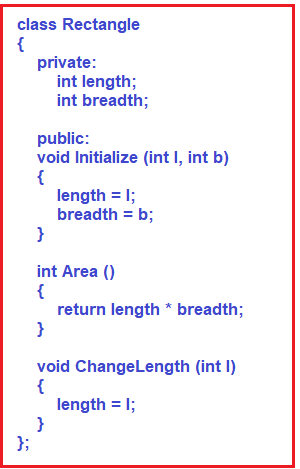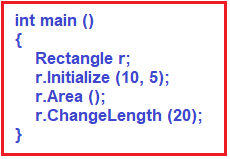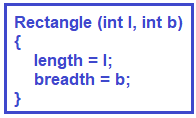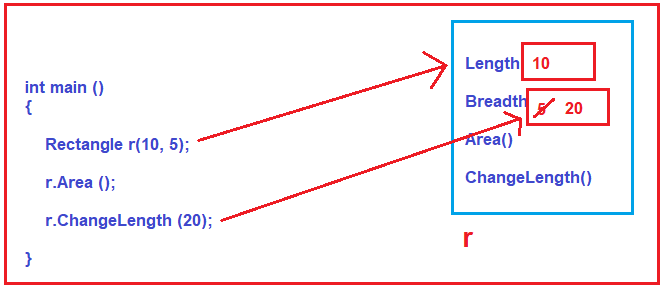# Converting a C program to a C++

## Converting a C program to a C++

In this article, we are going to discuss how to Convert a C program to a C++ Program. So, we are going to discuss the concepts that are useful in this course i.e. classes and constructors, not all the features of object-orientation. We will not be discussing everything about OOPS. We are going to Convert a C Program code to C++ code. The example that we discussed in our previous article, will try to convert to C++ code.

##### Example in C:

The following example we discussed in our previous article.

```#include <stdio.h>
struct Rectangle
{
int length;
};

void Initialize (struct Rectangle *p, int l, int b)
{
p->length = l;
}

int Area (struct Rectangle r)
{
}

void ChangeLength (struct Rectangle *p, int l)
{
p->length = l;
}

int main ()
{
struct Rectangle r;
Initialize (&r, 10, 5);
Area (r);
ChangeLength (&r, 20);
}
```

Here, we have a structure called Rectangle having length and breadth. Inside the main function, we are creating or declaring a variable of type Rectangle. You can see, inside the main function, there is no processing involved i.e. there are no operations, just function calls are there. So, the function call is for Initializing a Rectangle, finding the area of a rectangle, and changing its length, and all these functions we are passing rectangle as a parameter. All these functions (Initialize, Area, and ChangeLength) are related to the Rectangle. This is the best style of coding in the C language.

Now, let us see how to convert the above c language code into the C++ language code.

##### Converting Rectangle Structure to a C++ Class:

First, we need to convert the struct keyword with the class keyword and once you do this, then the Rectangle becomes a class. All these functions such as Initialize, Area, and ChangeLength are meant for the Rectangle only. So, in the second step, we need to move all these members inside the class only. The C++ class contains data members as well as functions. The functions Initialize, Area, and ChangeLength can access the rectangle class data members directly so there is no need to pass the Rectangle object as a parameter to these functions anymore. These functions can access the length and breadth member of the Rectangle directly. The last change that we need to do is, within the class Rectangle is, adding private keyword with a colon before data members and public keyword with a colon before the functions. For better understanding, please have a look at the following image.##### How it affects the main function?

Inside the main function, we need to create a variable of type Rectangle and then we need to call the methods using the same Rectangle variable as shown in the below image. If you observe now, we are not passing the Rectangle variable while calling the methods instead we are calling the methods using the rectangle variable and dot (.) operator.##### The complete code is given below:
```#include <iostream>
using namespace std;
class Rectangle
{
private:
int length;

public:
void Initialize (int l, int b)
{
length = l;
}

int Area ()
{
}

void ChangeLength (int l)
{
length = l;
}
};

int main ()
{
Rectangle r;
r.Initialize (10, 5);
r.Area ();
r.ChangeLength (20);
}
```
##### Constructor:

The Initialize function is used to initialize the data members i.e. length and breadth of the Rectangle. We need this function because the data members are private and we cannot access these two data members directly from outside of the Rectangle class i.e. we cannot access these two data members from the main function.

When we declare or create an object or declare a variable, at that time only we prefer initializing. That means instead of calling the Initialize function to initialize the data members, we need to initialize the data member at the time variable creation only. This is possible with the heap of a constructor. For that, we need to create a constructor within the Rectangle class as shown below. Please note the Constructor name must be the same as the class name and moreover, the constructor function should not have any return type even void.Now inside the main function, while creating a variable of type Rectangle we need to pass the length and breadth variable as shown below.

Rectangle r(10, 5);

With the above constructor in place, now we don’t require the Initialize function anymore to initialize the data members. The complete code is given below.

```#include <iostream>
using namespace std;
class Rectangle
{
private:
int length;

public:
Rectangle (int l, int b)
{
length = l;
}

int Area ()
{
}

void ChangeLength (int l)
{
length = l;
}
};

int main ()
{
Rectangle r(10, 5);
r.Area ();
r.ChangeLength (20);
}
```

Memory representation is given below.In the next article, I am going to discuss the C++ class and Constructor in detail. Here, in this article, I try to explain how to Convert a C program to a C++ Program and I hope you enjoy this Converting a C program to a C++ Program article.